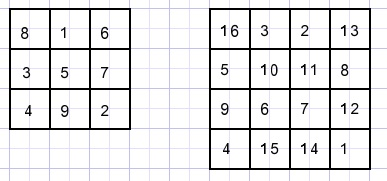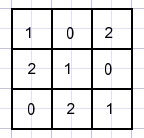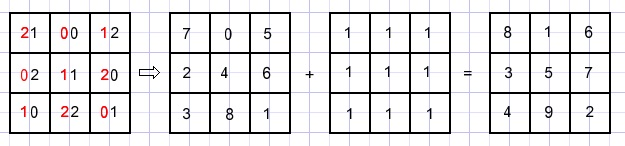Magic squares (1)Magic squares are tables of 3x3, 4x4, etc. with the numbers 1,2,3.. placed in their fields
such that all sums of rows, columns and diagonals are the same.
Below are two examples:What would this sum be in the case of a nxn square?
We know: 1 + 2 + 3 + ... + n = n(n+1)/2.
For a nxn square the sum of all fields is 1 + 2 + 3 + ...+ n2 = n2(1+n2)/2
The sum of one row(column, diagonal) is this value divided by n.
The sum is n(1 + n2)/2.

Magic squares always have raised great interest.
The 4x4 square pictured before is seen on an engraving made in 1514 by Dürer.
Note that 1514 is in the middle of the bottom row.How to make a magic square?
We have no proven way to start.
In such a situation a mathematician takes a step back and explorers a more simple case first.
We just want the sums equal, nothing more.
A square with only the numbers 1 does the job.
The next step is : fields of rows, columns, diagonals hold unique numbers 0,1,2.
So the sums are equal.
Below such a square is pictured:The problem is that numbers appear more than once in the square.
A tric fixes this problem.
Take the reflection around the middle column and combine the digits:Now all numbers are unequal and their sum is 33. Also the sequence is not 1,2,3... .
Or not?
Regard the numbers as written in number base 3, so multiply the left digit by 3 and add the right digit.
This results in the numbers 0,1,2,3,4,5,6,7,8. Almost OK.
Finally add 1 to each field and we have completed the square.A different approach.
We start from an empty 3x3 square.
We call the fields a,b,c,d,e,f,g,h,i and write the system of equations.``` a + b + c = 15 d + e + f = 15 g + h + i = 15 a + d + g = 15 b + e + h = 15 c + f + i = 15 a + e + i = 15 c + e + g = 15 ```
The equations are written in the program LinEq which solves systems of equations.
However this system cannot be solved because there are 9 unknowns and only 8 equations.
But one outcome is that e = 5, the center field only can have the number 5.
Let's make a = 1.
Trying to solve the equations now shows besides e=5 that f + h = 2.
This is not possible because f and h cannot be both equal to 1.
The number 1 may not appear in a corner field. So take b = 1, which makes h = 9.
Left is the choice of 6 and 8 for fields a and c. Let's make a = 8.
The other numbers follow from these choices.

A fast method to generate n * n squares for odd n.to 4x4 squares